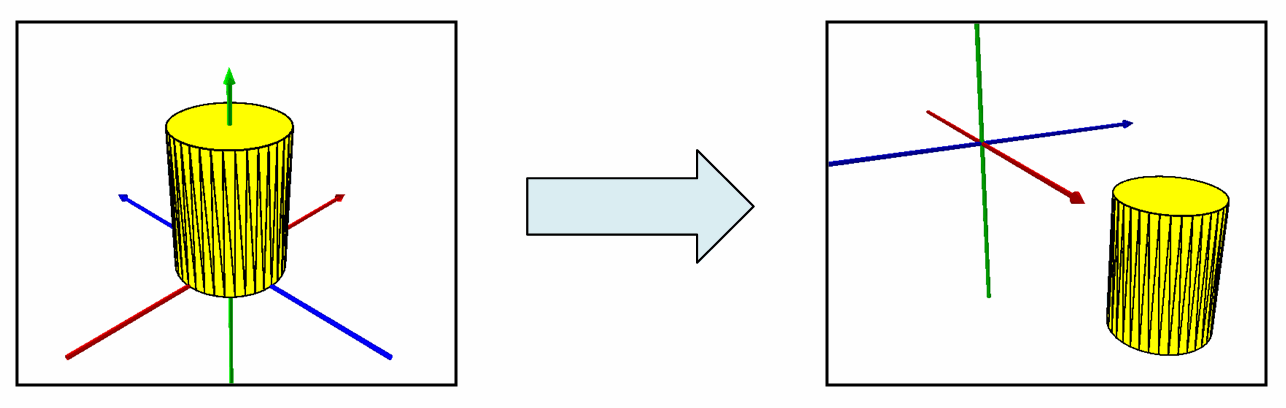ArcObjects Library Reference (Geometry)

# ITransform3D.MoveVector3D Method

Moves the object by an offset defined by a 3D vector.

```[Visual Basic .NET]
Public Sub MoveVector3D ( _
ByVal v As IVector3D _
)```
```[C#]
public void MoveVector3D (
IVector3D v
);```
```[C++]
HRESULT MoveVector3D(
IVector3D* v
);```
```[C++]
Parameters
v
v is a parameter of type IVector3D
```

#### Product Availability

Available with ArcGIS Engine, ArcGIS Desktop, and ArcGIS Server.
[C#]public static void TransformMultiPatchGeometry()

{

const double XComponent = 7.5;

const double YComponent = 7.5;

const double ZComponent = -10;

//Transform3D: Cylinder Repositioned Via MoveVector3D()

IVector3D vector3D = ConstructVector3D(
XComponent, YComponent, ZComponent
);

IGeometry geometry = GetMultiPatchGeometry();

ITransform3D transform3D = geometry as ITransform3D;

transform3D.MoveVector3D(vector3D);

}

private static IVector3D ConstructVector3D(double xComponent, double yComponent, double zComponent)

{

IVector3D vector3D = new Vector3DClass();

vector3D.SetComponents(xComponent, yComponent, zComponent);

return vector3D;

}